Ch 2. Derivatives I Multimedia Engineering Math DerivativeFormulas TrigonometricFunctions Differentiability ChainRule ImplicitDifferentiation
 Chapter 1. Limits 2. Derivatives I 3. Derivatives II 4. Mean Value 5. Curve Sketching 6. Integrals 7. Inverse Functions 8. Integration Tech. 9. Integrate App. 10. Parametric Eqs. 11. Polar Coord. 12. Series Appendix Basic Math Units Search eBooks Dynamics Fluids Math Mechanics Statics Thermodynamics Author(s): Hengzhong Wen Chean Chin Ngo Meirong Huang Kurt Gramoll ©Kurt GramollMATHEMATICS - CASE STUDY SOLUTION Although there are situations when a function cannot be differentiated, the discussion in this sectionfocuses on the function's differentiability. Differentiable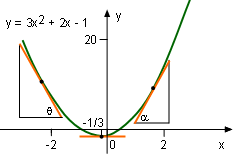y = 3x2 + 2x + 1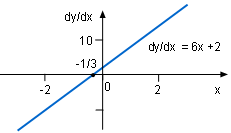dy/dx = 6x + 2 Consider for example, the function y = 3x2 + 2x - 1, then dy/dx = 6x + 2, which is reasonable. When x < -1/3, dy/dx or tanθ is negative and when x > -1/3, dy/dx or tanα is positive. When x= 1/3, the slope of the tangent line is horizontal and thus equal to 0. These correspond to its derivative function figure and it is obvious that the function is differentable at all points.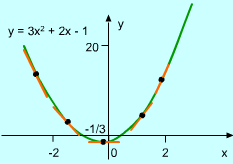3x2 + 2x - 1 is differentiable A function is differentiable at a point if the derivative of the function exists at that point. A function is differentiable on an interval if it is differentiable at every point in the interval, as already concluded. According to the above definition, y = 3x2 + 2x - 1 function is differentiable.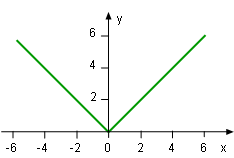y = (x2)0.5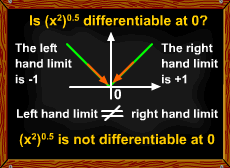(x2)0.5 is not Differentiable at all Points Now, consider another example, y = (x2)0.5. Is y differentiable in this case at all points? The definition of derivative gives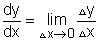In order to applying the definition to the y = (x2)0.5 function, Δy/Δx need to be find first. At x = 0,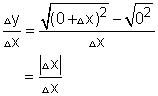The left hand limit is: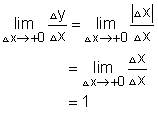The right hand limit is: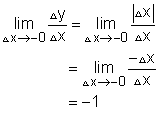The left hand limit is not equal to the right hand limit. Thus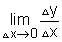does not exist, in other words, the function y = (x2)0.5 is not differentiable when x = 0. Differentiability and Continuity If a function is differentiable at point b, then this function is continuous at this specific point. proof: When x approaches b, the difference between f(x) and f(b) is yx - yb. Since x ≠ b, yx - yb can be written as: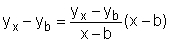Thus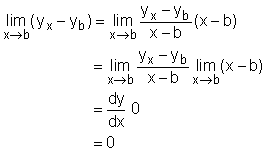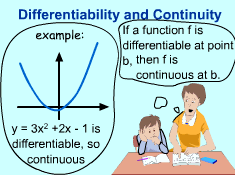Differentiability and Continuity I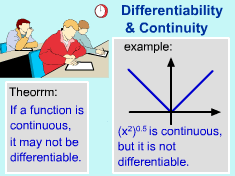Differentiability and Continuity II Therefore,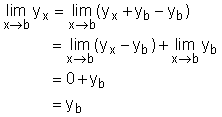This proves that when y is differentiable at point b, it is continuous at that point. Function y = 3x2 + 2x - 1 is differentiable in its variable range, and so it is continuous. If a function is continuous, is it differentiable? The answer is may not be. For example y = (x2)0.5 is continuous, but it was shown that it is not differentiable. Differentiability and Continuity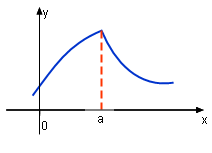Not Differentiable at a -- A Corner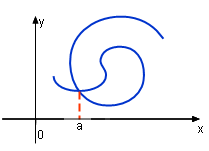Not Differentiable at a -- Kink or Loop Function y = (x2)0.5 is not differentiable at 0 because its left hand limit is not equal to its right hand limit. Normally, if a function's graphic has a corner or kink (loop), then the function is not differentiable.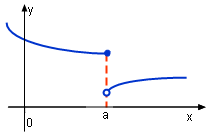Not Differentiable at a -- Function Discontinuous If a function's graphic is discontinuous, then this function is not differentiable. since the function's left and right hand limits are different.Not Differentiable at a -- Vertical Tangent Line When the curve of a continuous function has a vertical tangent line at point a, the slope of the tangent line is infinity. This means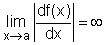Thus the function is not differentiable.